Note: You can hover cursor to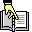to find the correct answer.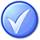0  (0%)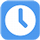6 Sec

1.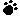Funding requirements for a project are usually in incremental amounts that are not continuous, and these appear as a step function in the graph depicting Cash flow, Cost baseline and Funding. Any gap at the end of the project, between funds allocated and the cost baseline and cash flow amounts represents:

A) The amount of Management Reserve that was not used
B) The amount of Management Reserve that was not allocated
C) The cost overrun
D) Ineffective utilization of funds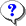Your answer=, Correct answer=, Work Sheet:

2.A project is estimated to cost \$ 50,000 with a timeline of 50 days. After 25 days, the project manager finds that 50% of the project is complete and Actual costs are \$ 50,000. What is the Cost Performance Index (CPI) ?

A) The CPI is 1
B) The CPI is 1.5
C) The CPI is 2
D) The CPI is 0.5Your answer=, Correct answer=, Work Sheet:

3.Lucy is a project manager involved in the Estimate Costs process in the initiation phase of a project. Given the limited detail available to her, what would you expect the range of her estimate to be and what would you call such an estimate?

A) -25 to +25 %, Rough Order of Magnitude
B) -10 to +10 %, Budgetary
C) -1 to +1 %, Definitive
D) -50 to +50 %, Rough Order of MagnitudeYour answer=, Correct answer=, Work Sheet:

4.Your project is mid-way through a delivery schedule. As project manager, you want to understand how much work is left. Which is the most accurate way to determine the remaining work to be done by the project team?

A) Rolling wave method
B) Earned Value Technique
C) A Manual forecast
D) Future analysisYour answer=, Correct answer=, Work Sheet:

5.An estimating technique that uses a statistical relationship between historical data and other variables (for example, square footage in construction, lines of code in software development) is known as:

A) Parametric Estimating
B) Analogous Estimating
C) Bottom-up Estimating
D) Historical AnalysisYour answer=, Correct answer=, Work Sheet:

 Click Turn Right Ahead sign for next test